Next: Energy Conservation Up: Traveling Waves Previous: Traveling Waves in an

# Wave Interference

What is the relationship between traveling wave and standing wave solutions to the wave equation, (361), in an infinite medium? To help answer this question, let us form a superposition of two traveling wave solutions of equal amplitude, and zero phase angle, that have the same wavenumber, but are moving in opposite directions. In other words,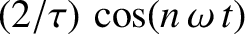(379)

Because the wave equation, (361), is linear, the previous superposition is a valid solution provided the two component waves are also valid solutions: that is, provided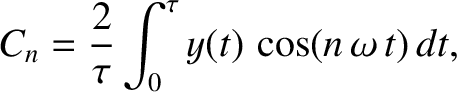, which we shall assume to be the case. Making use of the trigonometric identity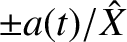(see Appendix B), the previous expression can also be written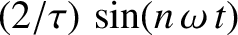(380)

which is a standing wave [cf., Equation (362)]. Evidently, a standing wave is a linear superposition of two, otherwise identical, traveling waves that propagate in opposite directions. The two waves completely cancel one another out at the nodes, which are situated at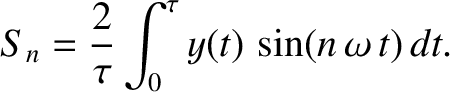, where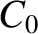is an integer. This process is known as total destructive interference. On the other hand, the waves reinforce one another at the anti-nodes, which are situated at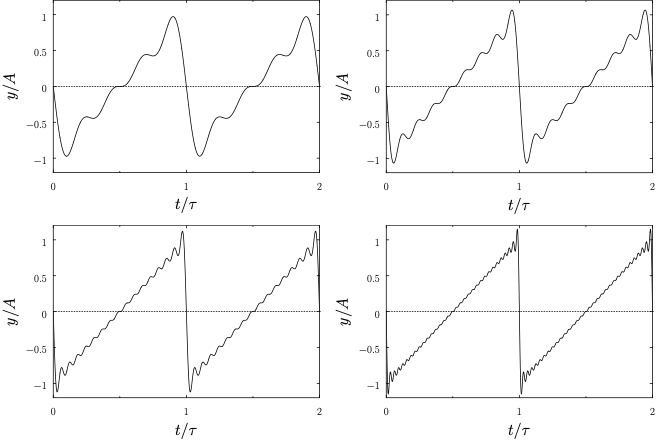, generating a wave whose amplitude is twice that of the component waves. This process is known as constructive interference.

As a more general example of wave interference, consider a superposition of two traveling waves of unequal amplitudes which again have the same wavenumber and zero phase angle, and are moving in opposite directions: that is,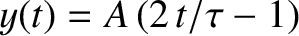(381)

where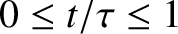. In this case, the trigonometric identitiesand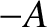(see Appendix B) yield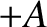(382)

Thus, the two waves interfere destructively at[i.e., at points where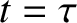and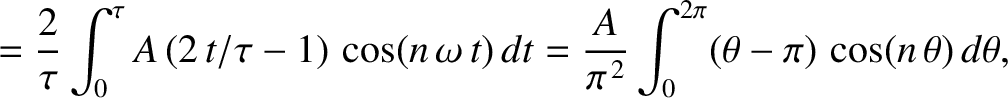] to produce a minimum wave amplitude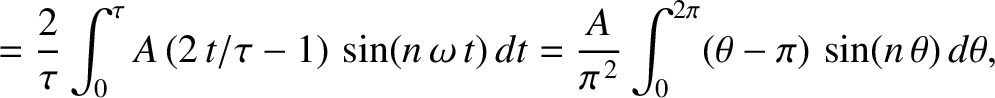, and interfere constructively at[i.e., at points where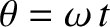and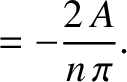] to produce a maximum wave amplitude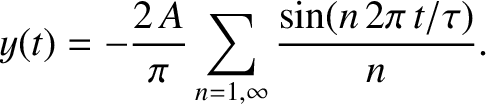. It can be seen that the destructive interference is incomplete unless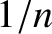. Incidentally, it is a general result that if two waves of amplitude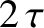and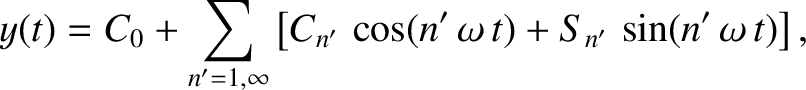interfere then the maximum and minimum possible values of the resulting wave amplitude areand, respectively.Next: Energy Conservation Up: Traveling Waves Previous: Traveling Waves in an
Richard Fitzpatrick 2013-04-08